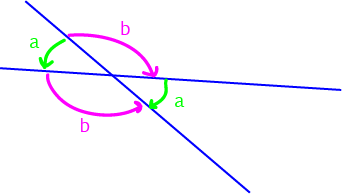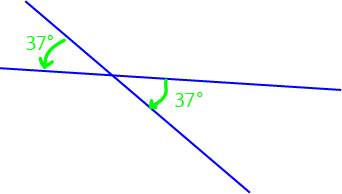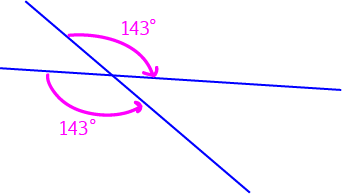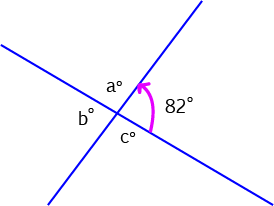Vertically Opposite Angles (vertical angles)When two lines cross, they form four different angles. These four angles can be grouped into two pairs of vertically opposite angles. One of these pairs is marked by green arrows (and the letter a) in the diagram, and the other is marked by pink arrows and the letter b. You can easily see why these angles are considered to be "opposite" each other, but you're probably wondering why they are "vertically" opposite each other. "Vertical" means up and down, doesn't it? This is even more curious when you realise that American books call these pairs of angles "vertical angles", forgetting about the "opposite" altogether.

Of course, living in the Southern Hemisphere, we are all too aware that "upwards" is a matter of opinion! But this isn't the reason for this curious name. Can you see how each pair of angles meets at a common point (corner)? This common point is called a vertex, and it is this "vertex" that gives us the "vertically" part of the term. The two angles in each pair are vertically opposite because they are on opposite sides of the same vertex.

Why All the Fuss About Vertically Opposite Angles?

The fact is, they're equal. This is a property of angles and lines that works regardless of whether the lines are parallel, perpendicular or what. In fact, if two lines cross to form vertically opposite angles, they are definitely not parallel! Let's look at some examples.

ExamplesIn the picture, the two angles marked with green arrows are vertically opposite angles. Since vertically opposite angles are equal, they both have the same size: $37^\circ$.In the picture, the two angles marked with pink arrows are vertically opposite angles. Since vertically opposite angles are equal, they both have the same size: $143^\circ$.

Now let's try an example like the ones in your maths text book.Exercise: Find all the unknown angles in the picture on the left.

Solution: First, the angle labelled with $b^\circ$ is vertically opposite the angle labelled with $82^\circ$. So, we must have $b^\circ = 82^\circ$.

Now we need to work out the remaining angles. But remember that a straight angle is equal to $180^\circ$. So, $a^\circ + 82^\circ = 180^\circ$, and $a^\circ = 180^\circ - 82^\circ = 98^\circ$.

Finally, $c^\circ = a^\circ$ since they are vertically opposite angles. Therefore, $c^\circ = 98^\circ$.

So, the answer is $a^\circ = 98^\circ$, $b^\circ = 82^\circ$ and $c^\circ = 98^\circ$.

Vertically opposite angles crop up all the time in geometry problems. It's a great idea to keep them in your geometry tool kit.

Description

In this mini book, you will learn about

1. Alternate angles
2. Cointerior angles
4. Vertically Opposite Angles

and several other topics related to lines and angles.

Audience

Year 10 or higher

Learning Objectives

To learn the basics of Lines and Angles stream of Geometry

Author: Subject Coach